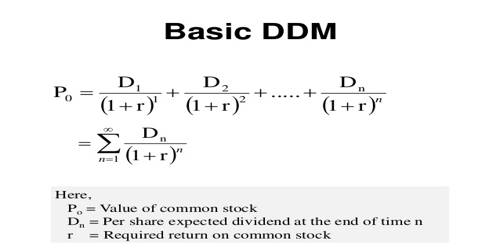# Dividend Discount Model (DDM)

Dividend Discount Model (DDM)

The dividend discount model (DDM) is a method of valuing a company’s stock price based on the theory that its stock is worth the sum of all of its future dividend payments, discounted back to their present value. In other words, it is used to value stocks based on the net present value of future dividends. is a quantitative method used for predicting the price of a company’s stock based on the theory that its present-day price is worth the sum of all of its future dividend payments. It is a method of valuing a company based on the theory that a stock is worth the discounted sum of all of its future dividend payments.

The equation most widely used is called the Gordon growth model. It is named after Myron J. Gordon of the University of Toronto, who originally published it along with Eli Shapiro in 1956 and made reference to it in 1959. Their work borrowed heavily from the theoretical and mathematical ideas found in John Burr Williams 1938 book “The Theory of Investment Value.” It is a method used to value stocks that use the theory that a stock is worth the sum of all of its future dividends.

The DDM calculates this present value in the following manner:

Present Stock Value = DividendShare / (RDiscount – RDividend Growth)

In the DDM, a present stock value that is higher than a stock’s market value indicates that the stock is undervalued and that it is a good time to purchase shares.